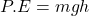## What is the force weight of a jaguar who jumps 3 meters to a tree branch with 2670 J of work?

Question

What is the force weight of a jaguar who jumps 3 meters to a tree branch with 2670 J of work?

in progress 0
2 months 2021-07-27T17:17:53+00:00 1 Answers 3 views 0

890 N

Explanation:

Given the following data;

Height = 3 m

Potential energy = 2670 J

To find the force weight;

We know that force = weight = mg

Potential energy can be defined as an energy possessed by an object or body due to its position.

Mathematically, potential energy is given by the formula;Where,

P.E represents potential energy measured in Joules.

m represents the mass of an object.

g represents acceleration due to gravity measured in meters per seconds square.

h represents the height measured in meters.

Substituting into the formula, we have;

2670 = mg*3

mg = 2670/3

mg = 890 N

Therefore, the force weight (mg) is equal to 890 Newton.